# Solution June 03, 2007

### Problem

i) Find all infinite arithmetic progressions of positive integers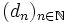$(d_n)_{n\in\mathbb{N}}$ such that dp is prime for all sufficiently large primes p.

ii) Find all polynomials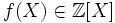$f(X) \in \mathbb{Z}[X]$ such that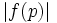$\left|f(p)\right|$ is prime for all sufficiently large primes p.

### Solution

i) is a special case of ii) so we will only solve ii) below.

Let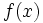$f(x)\,$ satisfy the condition in ii).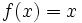$f(x) = x\,$ and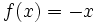$f(x) = -x\,$ are obviously solutions.

Assume that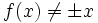$f(x)\neq \pm x$. Then there is a prime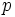$p\,$ with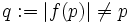$q:=|f(p)|\neq p\,$. Then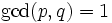$\gcd(p,q)=1\,$ so the sequence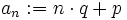$a_n:=n\cdot q+p$ contains infinitely many primes by Dirichlet's theorem (http://en.wikipedia.org/wiki/Dirichlet's_theorem_on_arithmetic_progressions).

Let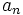$a_n\,$ be prime. Because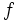$f\,$ is a polynomial with integer coefficients,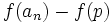$f(a_n)-f(p)\,$ is divisible by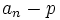$a_n-p\,$, which in turn is divisible by$q\,$ by the definition of$a_n\,$. We also have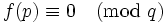$f(p)\equiv 0 \pmod q$ so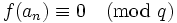$f(a_n)\equiv 0\pmod q$. But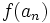$f(a_n)\,$ must be prime so that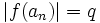$|f(a_n)| = q\,$. So$f(a_n)\,$ assumes one of the values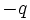$-q\,$ or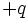$+q\,$ infinitely often which means that it is a constant polynomial.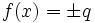$f(x) = \pm q$ for prime numbers$q\,$ are indeed solutions of ii). There are no other solutions.